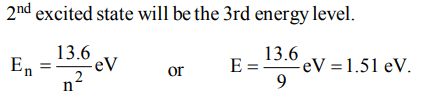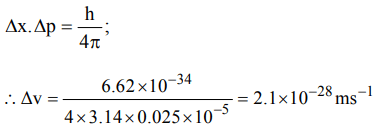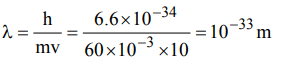## Atomic Structure Questions and Answers Part-15

1. Based on equation $E=– 2.178 × 10^{-18}J\left[\frac{Z^{2}}{n^{2}}\right]$    conclusions are written. Which of them is not correct ?
a) Larger the value of n, the larger is the orbit radius.
b) Equation can be used to calculate the change in energy when the electron changes orbit.
c) For n = 1, the electron has a more negative energy than it does for n = 6 which mean that the electron is more loosely bound in the smallest allowed orbit.
d) The negative sign in equation simply means that the energy or electron bound to the nucleus is lower than it would be if the electrons were at the infinite distance from the nucleus

Explanation: Energy of an electron at infinite distance from the nucleus is zero. As an electron approaches the nucleus, the electron attraction increases and hence the energy of electron decreases and thus becomes negative. Thus as the value of n decreases, i.e. lower the orbit is, more negative is the energy of the electron in it

2. In a hydrogen atom, if energy of an electron in ground state is 13.6. eV, then that in the 2nd excited state is
a) 1.51 eV
b) 3.4 eV
c) 6.04 eV
d) 13.6 eV.

Explanation:3. Uncertainty in position of a minute particle of mass 25 g in space is $10^{-5}m$   .What is the uncertainty in its velocity $\left( ms^{-1}\right)$  ? $\left(h = 6.6 * 10^{-34}Js\right)$
a) $2.1 * 10^{-34}$
b) $0.5 * 10^{-34}$
c) $2.1 * 10^{-28}$
d) $0.5 * 10^{-23}$

Explanation:4. The number of d-electrons retained in $Fe^{2+}$ (At. no. of Fe = 26) ion is
a) 4
b) 5
c) 6
d) 3

Explanation: Fe+2 = 3d6 , 4s0

5. The orbital angular momentum for an electron revolving in an orbit is given by $\sqrt{l\left(l+1\right)}\frac{h}{2\pi}$  . This momentum for an s-electron will be given by
a) zero
b) $\frac{h}{2\pi}$
c) $\sqrt{2}.\frac{h}{2\pi}$
d) $+\frac{1}{2}.\frac{h}{2\pi}$

Explanation: For s-electron, l = 0
angular momentum = zero

6. A reduction in atomic size with increase in atomic number is a characteristic of elements of
a) d-block
b) f-block
d) high atomic masses

Explanation: f-block elements show a regular decrease in atomic size due to lanthanide/actinide contraction.

7. Which one of the following groupings represents a collection of isoelectronic species ? (At. nos. : Cs : 55, Br : 35)
a) $N^{3-},F^{-},Na^{+}$
b) $Be,AI^{3+},Cl^{-}$
c) $Ca^{2+},Cs^{+},Br$
d) $Na^{+},Ca^{2+},Mg^{2+}$

Explanation: N3–, F and Na+ contain 10 electrons each.

8. In Bohr series of lines of hydrogen spectrum, the third line from the red end corresponds to which one of the following inter-orbit jumps of the electron for Bohr orbits in an atom of hydrogen
a) $5\rightarrow 2$
b) $4\rightarrow 1$
c) $2\rightarrow 5$
d) $3\rightarrow 2$

Explanation: The lines falling in the visible region comprise Balmer series. Hence the third line would be 1 n1 = 2, n2 = 5 i.e. 5 $\rightarrow$ 2

9. The de Broglie wavelength of a tennis ball of mass 60 g moving with a velocity of 10 metres per second is approximately Planck’s constant,$h = 6.63 × 10^{-34}Js$
a) $10^{-31}metres$
b) $10^{-16}metres$
c) $10^{-25}metres$
d) $10^{-33}metres$a) $n = 4, l = 3, m = + 1, s =+1\diagup2$
b) $n = 4, l = 4, m = -4, s =-1\diagup2$
c) $n = 4, l = 3, m = +4, s =+1\diagup2$
d) $n = 3, l =2 , m = -2, s =+1\diagup2$